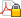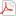Reference : Quadratization of symmetric pseudo-Boolean functions
 Document type : Scientific journals : Article Discipline(s) : Business & economic sciences : Quantitative methods in economics & managementPhysical, chemical, mathematical & earth Sciences : Mathematics To cite this reference: http://hdl.handle.net/2268/159368
 Title : Quadratization of symmetric pseudo-Boolean functions Language : English Author, co-author : Anthony, Martin [London School of Economics > > > >] Boros, Endre [Rutgers University (New Jersey) - RU > > > >] Crama, Yves[Université de Liège - ULiège > HEC-Ecole de gestion : UER > Recherche opérationnelle et gestion de la production >] Gruber, Aritanan [Rutgers University (New Jersey) - RU > > > >] Publication date : 2016 Journal title : Discrete Applied Mathematics Publisher : Elsevier Science Volume : 203 Pages : 1-12 Peer reviewed : Yes (verified by ORBi) Audience : International ISSN : 0166-218X City : Amsterdam Country : The Netherlands Keywords : [en] pseudo-Boolean function ; quadratic optimization ; symmetric function ; nonlinear integer programming Abstract : [en] A pseudo-Boolean function is a real-valued function $f(x)=f(x_1,x_2,\ldots,x_n)$ of $n$ binary variables; that is, a mapping from $\{0,1\}^n$ to ${\bbr}$. For a pseudo-Boolean function $f(x)$ on $\{0,1\}^n$, we say that $g(x,y)$ is a quadratization of $f$ if $g(x,y)$ is a Quadratic polynomial depending on $x$ and on $m$ auxiliary binary variables $y_1,y_2,\ldots,y_m$ such that $f(x)= \min \{ g(x,y) : y \in \{0,1\}^m \}$ for all $x \in \{0,1\}^n$. By means of quadratizations, minimization of $f$ is reduced to minimization (over its extended set of variables) of the quadratic function $g(x,y)$. This is of some practical interest because minimization of quadratic functions has been thoroughly studied for the last few decades, and much progress has been made in solving such problems exactly or heuristically. A related paper initiated a systematic study of the minimum number of auxiliary $y$-variables required in a quadratization of an arbitrary function $f$ (a natural question, since the complexity of minimizing the quadratic function $g(x,y)$ depends, among other factors, on the number of binary variables). In this paper, we determine more precisely the number of auxiliary variables required by quadratizations of \emph{symmetric} pseudo-Boolean functions $f(x)$, those functions whose value depends only on the Hamming weight of the input $x$ (the number of variables equal to 1). Research centres : QuantOM - HEC Management School Funders : Politique Scientifique Fédérale (Belgique) = Belgian Federal Science Policy Name of the research project : PAI COMEX Target : Researchers Permalink : http://hdl.handle.net/2268/159368 DOI : 10.1016/j.dam.2016.01.001

File(s) associated to this reference

Fulltext file(s):

FileCommentaryVersionSizeAccessRestricted access
quadofsymmetric.pdfVersion 1, December 2013Author preprint298.5 kBRequest copyOpen access
quadofsymmetric_WPULg_revised.pdfRevised version July 2015Author preprint147.95 kBView/OpenAll documents in ORBi are protected by a user license.